# 深度学习炼丹-数据预处理和增强

## 前言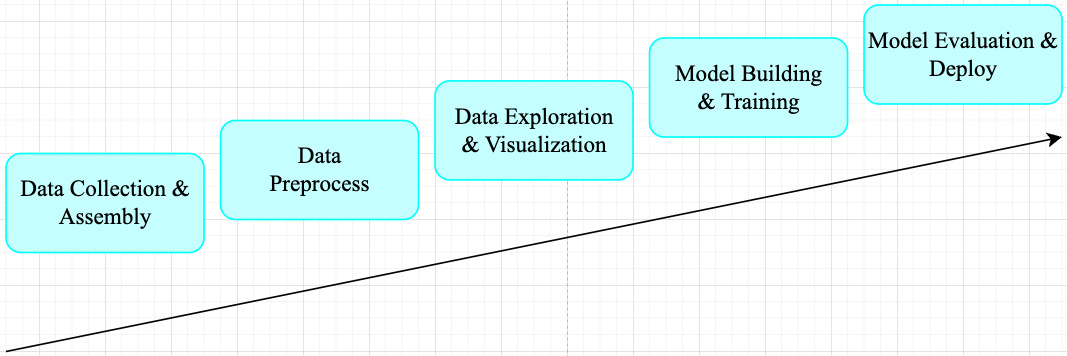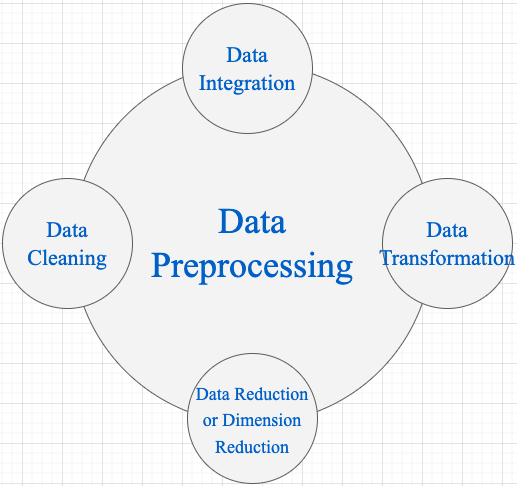1，Data Cleaning 数据清理是数据预处理步骤的一部分，通过填充缺失值、平滑噪声数据、解决不一致和删除​​异常值来清理数据。 2，Data Integration 用于将存在于多个源中的数据合并到一个更大的数据存储中，如数据仓库。例如，将来自多个医疗节点的图像整合起来，形成一个更大的数据库。 3，在完成 Data Cleaning 后，我们需要通过使用下述数据转换策略更改数据的值、结构或格式。 - Generalization: 使用概念层次结构将低级或粒度数据转换为高级信息。例如将城市等地址中的原始数据转化为国家等更高层次的信息。 - Normalization: 目的是将数字属性按比例放大或缩小以适合指定范围。Normalization 常见方法: - Min-max normalization - Z-Score normalization - Decimal scaling normalization

4，Data Reduction 数据仓库中数据集的大小可能太大而无法通过数据分析和数据挖掘算法进行处理。一种可能的解决方案是获得数据集的缩减表示，该数据集的体积要小得多，但会产生相同质量的分析结果。常见的数据缩减策略如下: - Data cube aggregation - Dimensionality reduction: 降维技术用于执行特征提取。数据集的维度是指数据的属性或个体特征。该技术旨在减少我们在机器学习算法中考虑的冗余特征的数量。降维可以使用主成分分析（PCA）等技术来完成。 - Data compression: 通过使用编码技术，数据的大小可以显着减小。 - Discretization: 数据离散化用于将具有连续性的属性划分为具有区间的数据。这样做是因为连续特征往往与目标变量相关的可能性较小。例如，属性年龄可以离散化为 18 岁以下、18-44 岁、44-60 岁、60 岁以上等区间。

## 一，Normalization 概述

### 1.1，Normalization 定义

Normalization 操作被用于对数据属性进行缩放，使其落在较小的范围之内（即变化到某个固定区间中），比如 [-1,1] 和 [0, 1]，简单理解就是特征缩放过程。很多机器学习算法都受益于 Normalization 操作，比如:

• 通常对分类算法有用。
• 在梯度下降等机器学习算法的核心中使用的优化算法很有用。
• 对于加权输入的算法（如回归和神经网络）以及使用距离度量的算法（如 K 最近邻）也很有用。

### 1.2，什么情况需要 Normalization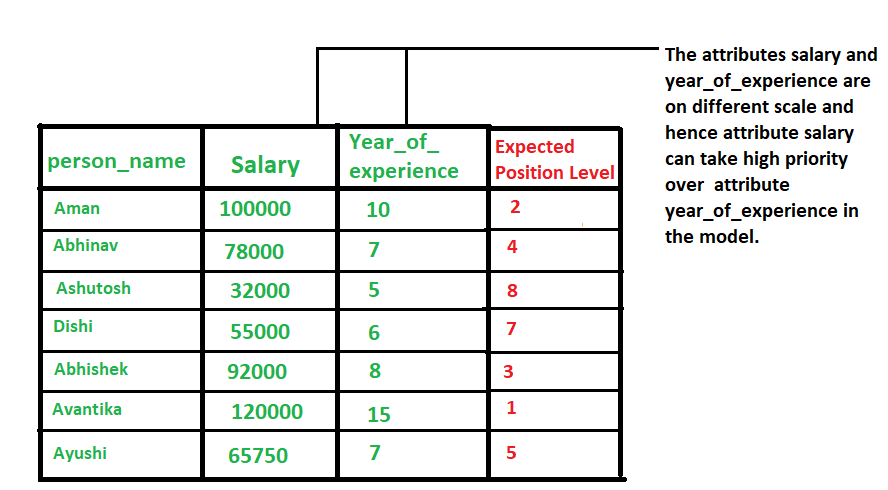### 1.3，Data Normalization 方法

1，z-Score Normalization

zero-mean Normalization，有时也称为 standardization，将数据特征缩放成均值为 0，方差为 1 的分布，对应公式:

$${x}' = \frac{x-mean(x)}{\sigma}$$

2，Min-Max Normalization

$${x}' = \frac{x - min(x)}{max(x) - min(x)}$$

### 1.4，示例代码

1，以下是使用 Python 和 Numpy 库实现 Min-Max Normalization 的示例代码：

python

# 导入必要的库

import numpy as np

# 定义数据集

X = np.array([[1, 2, 3], [4, 5, 6], [7, 8, 9]])

# 计算数据集的最小值和最大值

Xmin = X.min() Xmax = X.max()

# 计算最小-最大规范化

X_norm = (X - Xmin) / (Xmax - Xmin)

# 打印结果

print(X_norm)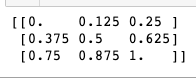## 二，normalize images

### 2.1，图像 normalization 定义

1，以单张图像的 zero-mean Normalization 为例，它使得图像的均值和标准差分别变为 0.01.0。因为是多维数据，与纯表格数据不同，它首先需要从每个输入通道中减去通道平均值，然后将结果除以通道标准差。因此可定义两种 normalization 形式如下所示:

shell

# min-max Normalization

output[channel] = (input[channel] - min[channel]) / (max[channel] - min[channel])

# zero-mean Normalization

output[channel] = (input[channel] - mean[channel]) / std[channel] 

### 2.3，PyTorch 实践图像 normalization

Pytorch 框架中，图像变换（image transformation）是指将图像像素的原始值改变为新值的过程。其中常见的 transformation 操作是使用 torchvision.transforms.ToTensor() 方法将图像变换为 Pytorch 张量（tensor），它实现了将像素范围为 [0, 255] 的 PIL 图像转换为形状为（C,H,W）且范围为 [0.0, 1.0] 的 Pytorch FloatTensor。另外，torchvision.transforms.normalize() 方法实现了逐 channel 的对图像进行标准化（均值变为 0，标准差变为 1）。总结如下:

• min-max Normalization: 对应 torchvision.transforms.ToTensor() 方法
• zero-mean Normalization: 对应 torchvision.transforms.Normalize() 方法，利用用均值和标准差对张量图像进行 zero-mean Normalization。

ToTensor() 函数的语法如下: shell """ Convert aPIL Imageornumpy.ndarray to tensor. Converts a PIL Image or numpy.ndarray (H x W x C) in the range [0, 255] to a torch.FloatTensor of shape (C x H x W) in the range [0.0, 1.0].

Args: pic (PIL Image or numpy.ndarray): Image to be converted to tensor. Returns: Tensor: Converted image. """ 

Normalize() 函数的语法如下:

shell Syntax: torchvision.transforms.Normalize()

Parameter: * mean: Sequence of means for each channel. * std: Sequence of standard deviations for each channel. * inplace: Bool to make this operation in-place. Returns: Normalized Tensor image. 

# import necessary libraries

from PIL import Image import matplotlib.pyplot as plt import numpy as np import torchvision.transforms as transforms import matplotlib.pyplot as plt

def show_images(imgs, num_rows, num_cols, titles=None, scale=8.5): """Plot a list of images.

Defined in :numref:sec_utils"""
figsize = (num_cols * scale, num_rows * scale)
_, axes = plt.subplots(num_rows, num_cols, figsize=figsize)
axes = axes.flatten()
for i, (ax, img) in enumerate(zip(axes, imgs)):
try:
img = np.array(img)
except:
pass
ax.imshow(img)
ax.axes.get_xaxis().set_visible(False)
ax.axes.get_yaxis().set_visible(False)
if titles:
ax.set_title(titles[i])
return axes


def normalize_image(image_path): img = Image.open(img_path) # load the image # 1, use ToTensor function transform = transforms.Compose([ transforms.ToTensor() ]) img_tensor = transform(img) # transform the pIL image to tensor # 2, calculate mean and std by tensor's attributes mean, std = img_tensor.mean([1,2]), img_tensor.std([1,2]) # 3, use Normalize function transform_norm = transforms.Compose([ transforms.ToTensor(), transforms.Normalize(mean, std) ]) img_normalized = transform_norm(img) # get normalized image

img_np = np.array(img) # convert PIL image to numpy array
# print array‘s shape mean and std
print(img_np.shape) # (height, width, channel), (768, 1024, 3)
print("mean and std before normalize:")
print("Mean of the image:", mean)
print("Std of the image:", std)
return img_np, img_tensor, img_normalized


def convert_tensor_np(tensor): img_arr = np.array(tensor) img_tr = img_arr.transpose(1, 2, 0) return img_tr

if name == 'main': img_path = 'Koalainputimage.jpeg' img_np, img_tensor, img_normalized = normalize_image(img_path) # transpose tensor to numpy array and shape of (3,,) to shape of (,,3) img_normalized1 = convert_tensor_np(img_tensor) img_normalized2 = convert_tensor_np(img_normalized) show_images([img_np, img_normalized1, img_normalized2], 1, 3, titles=["orignal","min-max normalization", "zero-mean normalization"]) 

1，程序输出和两种 normalization 操作效果可视化对比图如下所示: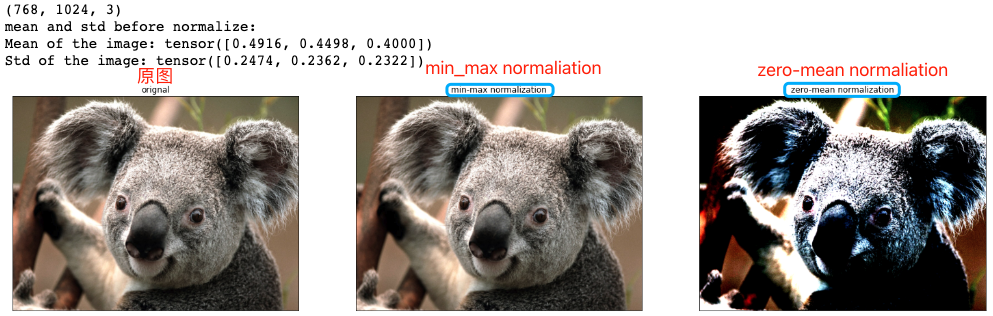2，原图和两种 normalization 操作后的图像像素值分布可视化对比图如下所示: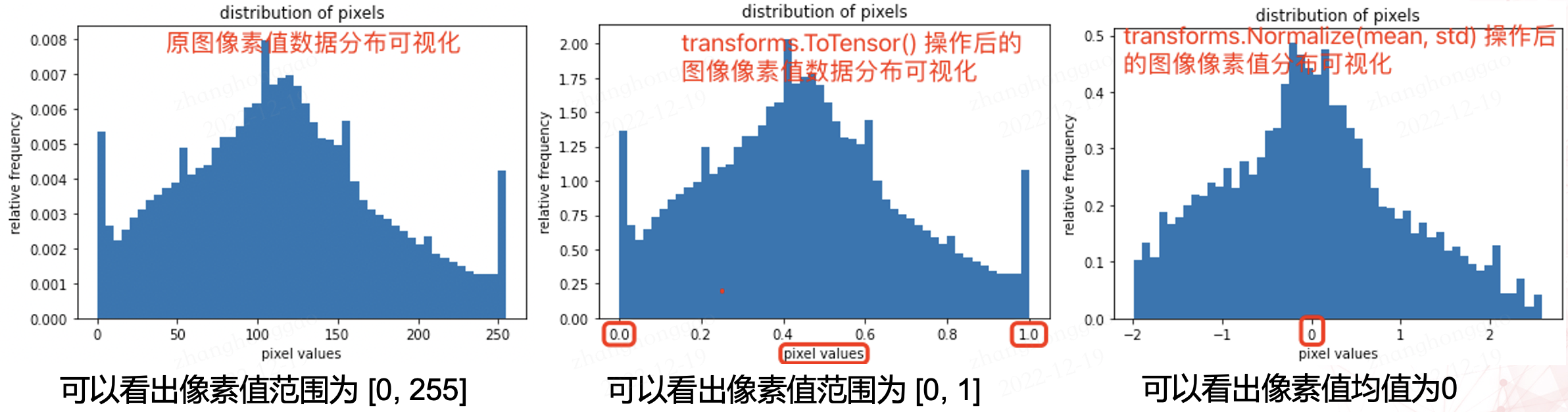python

# plot the pixel values

plt.hist(img_np.ravel(), bins=50, density=True) plt.xlabel("pixel values") plt.ylabel("relative frequency") plt.title("distribution of pixels") 

## 三，数据增强(扩增)

HSV 表达彩色图像的方式由三个部分组成： Hue（色调、色相） Saturation（饱和度、色彩纯净度） Value（明度）

### 3.1，opencv 图像处理

#### 图像的几何变换

OpenCV 提供的几何变换函数如下所示:

1，拓展缩放: 拓展缩放，改变图像的尺寸大小

cv2.resize(): 。常用的参数有设定图像尺寸、缩放因子和插值方法。

2，平移: 将对象换一个位置。

cv2.warpAffine(): 函数第一个参数是原图像，第二个参数是移动矩阵，第三个参数是输出图像大小 (width,height)。举例，如果要沿 $(x，y)$ 方向移动，移动的距离是 $(tx ，ty)$，则以下面的方式构建移动矩阵: $$\begin{bmatrix} 1 & 0 & t_x\ 0 & 1 & t_y \end{bmatrix}$$

3，旋转: 对一个图像旋转角度 $\theta$。

getRotationMatrix2D 函数第一个参数为旋转中心，第二个为旋转角度，第三个为旋转后的缩放因子

4，放射变换（也叫平面变换/径向变换）: 在仿射变换中，原图中所有的平行线在结果图像中依旧平行。

5，透视变换（也叫空间变换）: 转换之后，直线仍是直线。

python import cv2 import matplotlib.pyplot as plt from PIL import Image import numpy as np

# Some Geometric Transformation of Images

class GeometricTransAug(object): def init(self, image_path): img = Image.open(image_path) # load the image self.img_np = np.array(img) # convert PIL image to numpy array self.rows, self.cols, self.ch = self.img_np.shape self.geometry_trans_aug_visual(self.img_np)

def resize_aug(self, img_np):
# 直接设置了缩放因子, 缩放原大小的2倍
res = cv2.resize(img_np, None, fx=2, fy=2, interpolation = cv2.INTER_CUBIC)
return res

def warpAffine_aug(self, img_np):
# 先构建转换矩阵, 将图像像素点整体进行(100,50)位移：
M = np.float32([[1,0,100],[0,1,50]])
res = cv2.warpAffine(img_np, M,(self.cols, self.rows))
return res

def rotation_aug(self, img_np):
rows, cols, ch = img_np.shape
# 先构建转换矩阵,图像相对于中心旋转90度而不进行任何缩放。
M = cv2.getRotationMatrix2D((self.cols/2, self.rows/2), 90, 1)
res = cv2.warpAffine(img_np, M, (self.cols, self.rows))
return res

# 仿射变换需要从原图像中找到三个点以及他们在输出图像中的位置
pts1 = np.float32([[50,50],[200,50],[50,200]])
pts2 = np.float32([[10,100],[200,50],[100,250]])
# 通过 getAffineTransform 创建一个 2x3 的转换矩阵
M = cv2.getAffineTransform(pts1,pts2)

res = cv2.warpAffine(img_np, M, dsize = (self.cols, self.rows))
return res

def perspective_trans_aug(self, img_np):
# 透视变换需要一个 3x3 变换矩阵
pts1 = np.float32([[56,65],[368,52],[28,387],[389,390]])
pts2 = np.float32([[0,0],[300,0],[0,300],[300,300]])

M = cv2.getPerspectiveTransform(pts1,pts2)
# dsize: size of the output image.
res = cv2.warpPerspective(img_np, M, dsize = (300,300))

return res

def geometry_trans_aug_visual(self, img_np):
res1 = self.resize_aug(img_np)
res2 = self.warpAffine_aug(img_np)
res3 = self.rotation_aug(img_np)
res5 = self.perspective_trans_aug(img_np)
imgs = [res1, res2, res3, res4, res5]
aug_titles = ["resize_aug", "warpAffine_aug", "rotation_aug", "radial_trans_aug", "perspective_trans_aug"]
# show_images 函数前文已经给出，这里不再复制过来
axes = show_images(imgs, 2, 3, titles=aug_titles, scale=8.5)


if name == 'main': img_path = 'Koalainputimage.jpeg' geometry_trans_aug = GeometricTransAug(img_path) img_np2 = geometry_trans_aug.img_np print(img_np2.shape)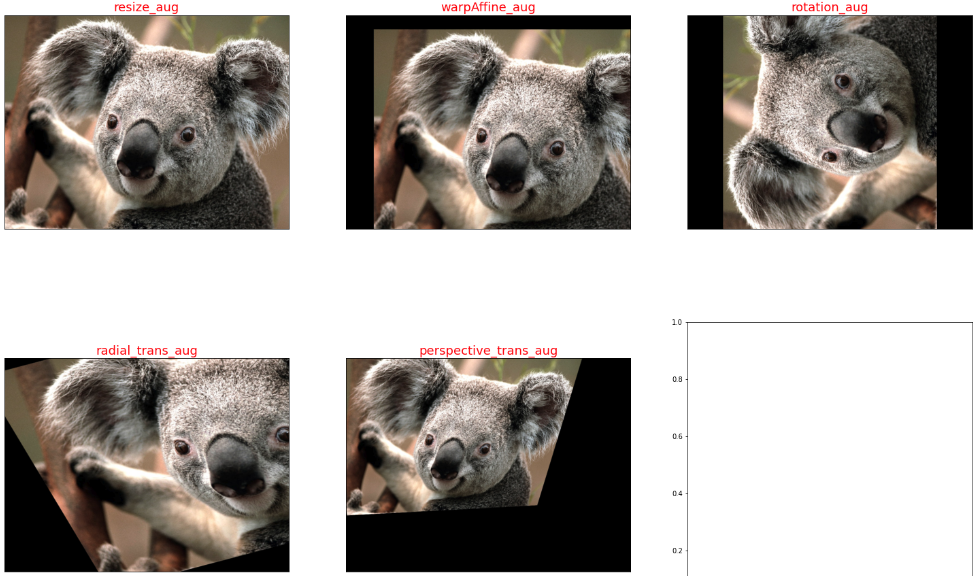### 3.2，pytorch 图像增强

pytorch 框架中，transforms 类提供了 22 个数据增强方法，对应代码在 transforms.py 文件中，它们既可以对 PIL Image 也能对 torch.*Tensor 数据类型进行增强。

api 的详细介绍可以参考官网文档-Transforming and augmenting images。本章只对 transforms 的 22 个方法进行简要介绍和总结。

1，裁剪-Crop

• 中心裁剪: transforms.CenterCrop
• 随机裁剪: transforms.RandomCrop
• 随机长宽比裁剪: transforms.RandomResizedCrop
• 上下左右中心裁剪: transforms.FiveCrop
• 上下左右中心裁剪后翻转: transforms.TenCrop

2，翻转和变换-Flip and Rotations

• 依概率 p 水平翻转:transforms.RandomHorizontalFlip(p=0.5)
• 依概率 p 垂直翻转:transforms.RandomVerticalFlip(p=0.5)
• 随机旋转:transforms.RandomRotation

3，图像变换

• resize: transforms.Resize
• min-max Normalization: 对应 torchvision.transforms.ToTensor() 方法
• zero-mean Normalization: 对应 torchvision.transforms.Normalize() 方法
• 修改亮度、对比度和饱和度:transforms.ColorJitter
• 转灰度图: transforms.Grayscale
• 线性变换: transforms.LinearTransformation()
• 仿射变换: transforms.RandomAffine
• 依概率 p 转为灰度图: transforms.RandomGrayscale
• 将数据转换为 PILImage: transforms.ToPILImage
• transforms.Lambda: Apply a user-defined lambda as a transform.

4，对 transforms 操作，使数据增强更灵活

• transforms.RandomChoice(transforms): 从给定的一系列 transforms 中选一个进行操作
• transforms.RandomApply(transforms, p=0.5): 给一个 transform 加上概率，依概率进行操作
• transforms.RandomOrder: 将 transforms 中的操作随机打乱

python

# 为了节省空间，这里不再列出导入相应库的代码和show_images函数

img_PIL = Image.open('astronaut.jpeg') print(img_PIL.size)

# properly show that the image can be both transformed and not transformed!

torch.manual_seed(0)

# size 参数: desired output size.

resized_imgs = [transforms.Resize(size=size)(orig_img) for size in (30, 50, 100, orig_img.size)] show_images(resized_imgs, 1, 4)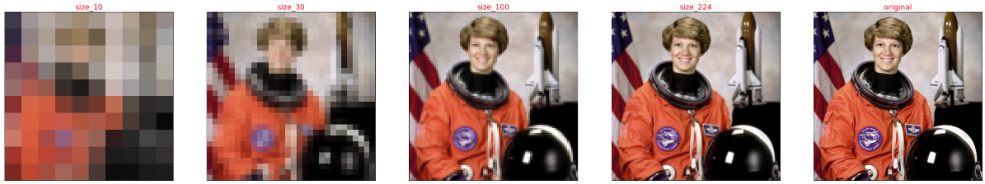### 3.3，imgaug 图像增强

imgaug 是一个用于机器学习实验中图像增强的库。 它支持广泛的增强技术，允许轻松组合这些技术并以随机顺序或在多个 CPU 内核上执行它们，具有简单而强大的随机接口，不仅可以增强图像，还可以增强关键点/地标、边界框、 热图和分割图。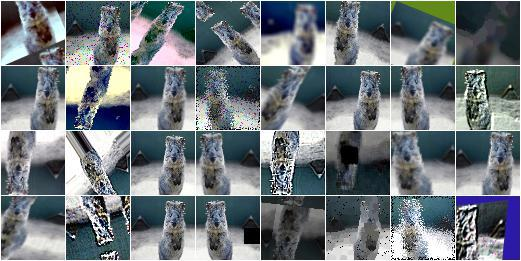imgaug 的图像增强方法如下所示。

• Basics
• Keypoints
• Bounding Boxes
• Heatmaps